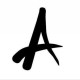Geometry

# Geometry Problems of RMO’17

Q.1. Let AOB be a given angle less than$180^{\circ}$ and let P be an interior point of the angular region determined by$\angle AOB$. Show, with proof, how to construct, using only ruler and compass, a line segment CD passing through P such that C lies on the way OA and D lies on the ray OB, and CP:PD=1:2.Analysis: Draw a line parallel to OB through C. Let it intersect OP (extended) at K.$\triangle CPK$ and$\triangle DPO$ are similar which implies$OP : PK = DP : PC = 2:1.$

Construction: Choose K on extended OP such that OP : PK=2:1. Draw a line parallel to OB through K. It will intersect OA at C (required). Then CP will cut OB at D (required).

Q.5. Let$\Omega$ be a circle with a chord AB which is not a diameter.$\Gamma_{1}$ be a circle on one side of AB such that it is tangent to AB at C and internally tangent to$\Omega$ at D. Likewise, let$\Gamma_{2}$ be a circle on the other side of AB such that it is tangent to AB at E and internally tangent to$\Omega$ at F. Suppose the line DC intersects$\Omega$ at$X \neq D$ and the line FE intersects$\Omega$ at$Y \neq F$. Prove that XY is a diameter of$\Omega.$Label the centres as P and Q, as shown in the figure. Let O be the centre of the main circle.$\angle PCD=\angle PDC=\angle OXD$ gives$XO\parallel CP.$ Now,$CP\bot AB,$ so we get$XO\bot AB.$ Thus XO is nothing but the perpendicular bisector of AB. Similarly YO is also the perpendicular bisector of AB. Hence X, O, Y are collinear. Hence done.

## 4 thoughts on “Geometry Problems of RMO’17”

1.Shubhav Jain says:

What does this mean:
\$XO\bot AB.\$

Liked by 1 person

1.Aditya Ghosh says:

That was just a latex command. I’ve fixed it now.

Liked by 1 person

2.Shubhav Jain says:

Why:
Thus XO is nothing but the perpendicular bisector of AB.

Liked by 1 person

1.Shubhav Jain says:

Got it!!!

Liked by 1 person